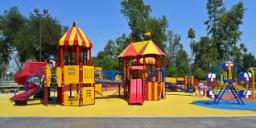# Rectangular 5611

The rectangular course is 12 m longer than its width. Suppose its length increases by 10 m and its area increases by 600 square meters. What are its dimensions?

a =  72 m
b =  60 m

### Step-by-step explanation:Did you find an error or inaccuracy? Feel free to write us. Thank you!

Tips for related online calculators
Are you looking for help with calculating roots of a quadratic equation?
Do you have a linear equation or system of equations and looking for its solution? Or do you have a quadratic equation?
Do you want to convert length units?

#### Grade of the word problem:

We encourage you to watch this tutorial video on this math problem: